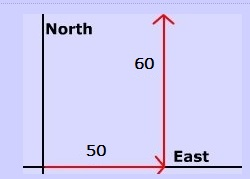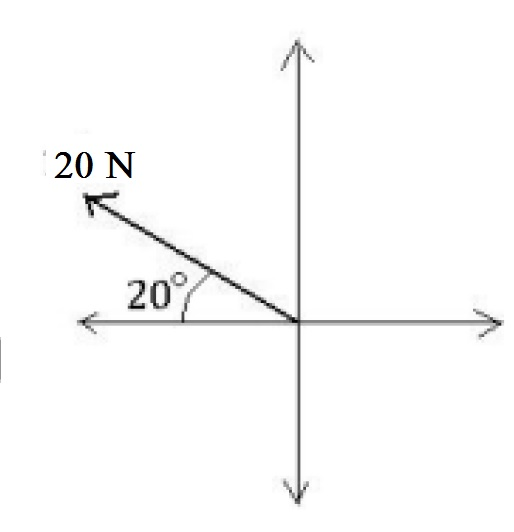# Practice on Force and Density

Question 1: As per the figure below, find he magnitude of the resultant vector.• 64
• 66
• 75
• 78
Question 2: What will be the direction of the resultant vector in the figure above (question 1)?

• north east
• north west
• south east
• north west
Question 3: As per the figure below, find the horizontal component of the force.• 18.8 N
• − 18.8 N
• 19.0
• − 19.0 N
Question 4: As per the figure above (question 3), find the vertical component of the force.
• 7 N
• − 6.84 N
• 6.84 N
• 9 N
Question 5: Assume that a body of mass of 50 g and a volume of 2 cm3.
Find the item's density in kg/m3.

• 27,000 kg/m3
• 25,000 kg/m3
• 25 k/cm3
• 25,000 g/m3